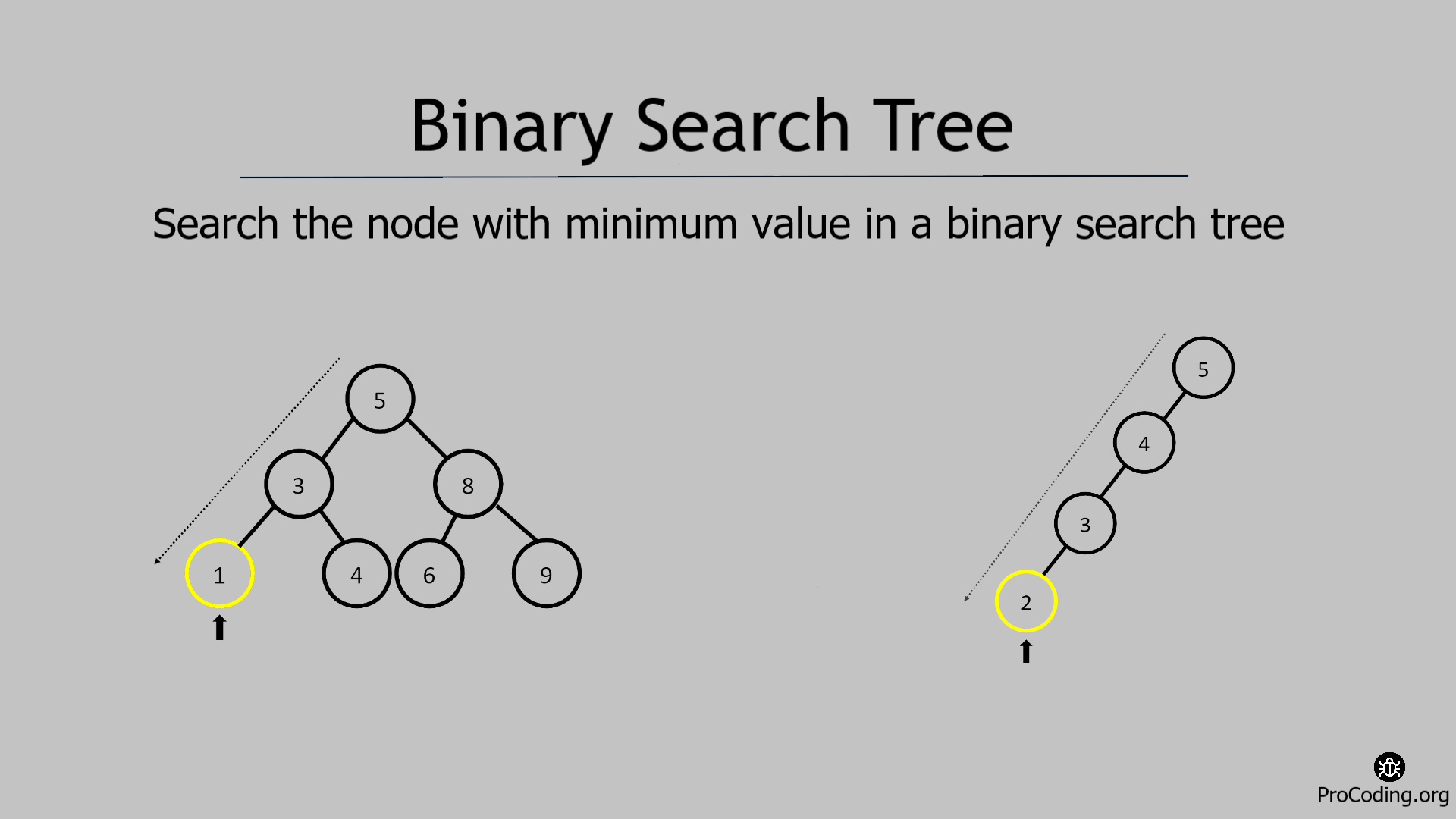# Search the node with minimum value in a Binary search tree

Abhishek Kumar Category: Data StructuresTags: datastructures binary-search-tree

Search the node with minimum value in a binary search tree.
To search a node with a minimum value in a Binary Search Tree, we have to traverse to the left-most node at the bottom.To search a node with minimum value in a Binary Search Tree (BST), we have to traverse to the left-most node at the bottom.

Because, in BST small element is always on the left side so minimum element will be location on the left-most at the bottom.

##### Example -
```Input:
5
/   \
3     8
/ \   / \
1   4 6   9

Output: 1```

We can solve this problem in two ways-

### Recursive solution

###### Algorithm
Find_Min(root)
``````1.  if root is null then return null
2.  if root.left is null then return root.data
3.  else return Find_Min(root.left)``````
``````class Node:
def __init__(self, data):
self.left = None
self.data = data
self.right = None

def find_min(root):
if root is None:
return None
if root.left is None:
return root.data
else:
return find_min(root.left)

bst_root = Node(10)
bst_root.left = Node(6)
bst_root.right = Node(16)
bst_root.left.left = Node(4)
bst_root.left.right = Node(9)
bst_root.right.left = Node(13)
bst_root.right.right = Node(20)
print(find_min(bst_root))``````

Output

`4`

### Iterative solution

###### Algorithm
Find_Iter(root)
``````1.  if root is null then return null
2.  node = root
3.  while node.left
4.      node = node.left
5.  return node.data``````
``````class Node:
def __init__(self, data):
self.left = None
self.data = data
self.right = None

def find_min(root):
if root is None: return None
node = root
while node.left:
node = node.left
return node.data

bst_root = Node(10)
bst_root.left = Node(6)
bst_root.right = Node(16)
bst_root.left.left = Node(4)
bst_root.left.right = Node(9)
bst_root.right.left = Node(13)
bst_root.right.right = Node(20)
print(find_min(bst_root))``````

#### Output

Output

`4`

Time complexity: The time complexity of both of these solution will be O(log n) if the tree is balanced but in worst case the time complete will be O(n) if the tree is not balanced i.e, tree becomes skewed.

AUTHOR### Abhishek Kumar

Software engineer | Blogger | Keen learner
Python | Django | JavaScript | React | Next.js | Express.js | C
Passionate about learning and experimenting with new things and open to more opportunities and collaborations.